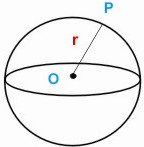HOME MATH DICTIONARY DOWNLOAD FEEDBACK DISCLAIMER
 Question: What is Sphere ? Answer: A sphere is a perfectly round shape. Objects which are in the shape of a sphere are called spherical. Balls, balloons and soap bubbles are often spherical. A sphere is defined as the set of point P in space which are all the same distance r from a fixed point O. O is the center of the sphere r is its radius.The area of the surface of the sphere is 4 π r2 The volume enclosed by the sphere is 4/3 π r3